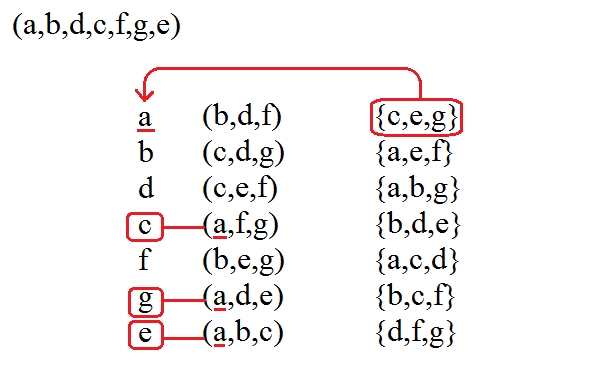None:
Polyps:
Strongs:RevelationThe First Trumpet We noted that Christ could open the last three seals because of His divine triune nature. The associations of the groups; e = [0,a,b,c] where unity, 'a' is corresponding to [0,b,d,f] g = [0,a,d,e] c = [0,a,f,g] was replaced with one in an alignment a = [0,a,b,c] where unity, 'a' is corresponding to [0,a,b,c] b = [0,a,d,e] c = [0,a,f,g] Which permit the opening of these last seals in the octal group with an alignment rather than a "reversal" (it opens up a whole new system.) For now we have two sets of associations with each seven cycle. (The left and right hands as before) and the left handed type is of a different octal whose elements [0,c,e,g] etc represent a K4 group (static from frobenius on the left handed octal here.) Using a seven cycle (a,b,d,c,f,g,e); a = [0,b,d,f] = [0,c,e,g] b = [0,c,d,g] = [0,a,e,f] d = [0,c,e,f] = [0,a,b,g] c = [0,a,f,g] = [0,b,d,e] f = [0,b,e,g] = [0,a,c,d] g = [0,a,d,e] = [0,b,c,f] e = [0,a,b,c] = [0,d,f,g] We also have another seven cycle for our original octal that corresponds to 'a' as unity with [0,b,d,f] held fixed under frobenius. Using (a,b,f,c,d,e,g) a = [0,b,d,f] = [0,c,e,g] b = [0,c,e,f] = [0,a,d,g] f = [0,c,d,g] = [0,a,b,e] c = [0,a,d,e] = [0,b,f,g] d = [0,b,e,g] = [0,a,c,f] e = [0,a,f,g] = [0,b,c,d] g = [0,a,b,c] = [0,d,e,f] Now the new associations in the right hand column are from two different (left-handed, confusing I know) octals; They have different operations. There is one shared group [0,c,e,g] which corresponds to identity in the right hand. Although the seven cycles used (with different unity) both preserve the groups of the middle (sun octal) column they do not preserve the groups of each other's right hand column. The groups are "opposed" to each other. We will identify this new right hand column by the term "fire" for the complement in these left handed groups. Since these two columns under these cycles may form groups under K4 subgroup addition (then they contain a singleton each - a common intersection), we will term the intersection as "blood". Then as these two sets (middle and right columns) do not agree, we will distinguish the two sets as "hail and fire mingled with blood" - the hail being the opposite of fire (i.e. hot/cold). We will consider the two sets hail and fire being cast upon the earth, or earth as representing the four beasts from which the cycles are generated. (we used [0,b,d,f] = a here, although [0,b,e,g] or [0,c,d,g] or [0,c,e,f] would also do.) These are in analogy the "four winds of heaven". The term 'blood' I do not relate specifically to the multiplicative identity - merely the intersection, as it is common to both left and right octals when groups of subgroups appear. The "blood" of each subgroup in either the hail/fire column associates the singletons in the seven cycle (here on the left) with subgroups of subgroups in the hail/fire column's companion octal. Thus we see a sort of circulatory property of these extra correspondences with these "hail" and "fire" groups. (The "blood" is related to the right hand element that would be the identity that holds that corresponding subgroup fixed under frobenius.)Then the alignment of "fire" is found in the singletons (green things - as if a "shoot") and K4 subgroups (trees - as if branching) under addition in the right hand of the octal. The left hand of triples align in the opposite direction as "hail". In that manner the green things and the trees (singletons and groups respectively) are "burned" upwards, against the direction of the hail. The hail and fire sets or "hot and cold " do not share a single K4 subgroup, or even as in alignment with the other column's groups. (So that the rows of each subgroup forms a subgroup in the companion's octal also) For they align with the right hand seven cycle or are in reverse alignment We will term this as "the third part" (product) of trees is burnt up. I.e. The association of groups of subgroups reverts to the intersection, or singleton in the seven cycle (the fire). The left handed octal is aligned to the "static" elements in the seven cycle whereas the right handed octal is opposed to it. (see the positions of subgroups in the columns above.) One is therefore "fire" and the other "hail". (fire burns up, hail falls down) The intersections of groups formed by "three group triples" (a product of trees) correspond to singletons and their companion either as from the left or the right - and they circulate "blood" between them. Likewise there is no "left hand octal" defined per se, rather just the sets of triples or "trees". The green grass - (shooting forth life) of singletons is "burned up" also, the operation within the left hand triples that would form the companion octal is "burned up", or equivalently the product of the original octal is not valid in the complement "octal". These "hail and fire" as cast upon the earth, what are they? If the four beasts about the throne are associated with the earth, then we see that this "hail and fire" is valid for the equivalent trumpets to the first four seals only, and not the last three. (then a = (b,d,f) etc, in the octal as legally!). The hail/fire columns' subgroups are aligned or reversed with the position of "static" groups in our right handed C7 elements though - which allows the transitions of the first four/last three seals to make sense here.Continue To Next Page Return To Section Start Return To Previous Page'